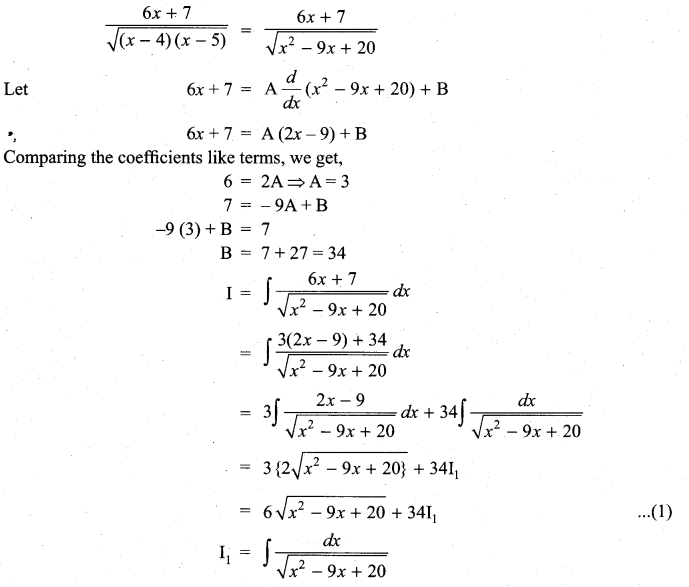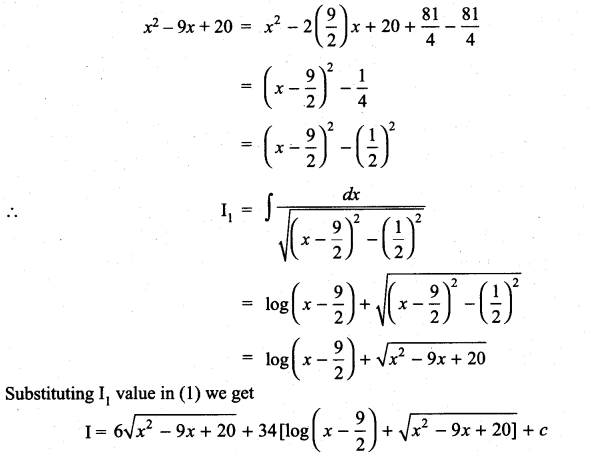# Samacheer Kalvi 11th Maths Solutions Chapter 11 Integral Calculus Ex 11.11

## Tamilnadu Samacheer Kalvi 11th Maths Solutions Chapter 11 Integral Calculus Ex 11.11

Integrate the following with respect to x:
Question 1.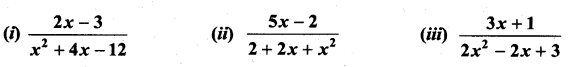Solution: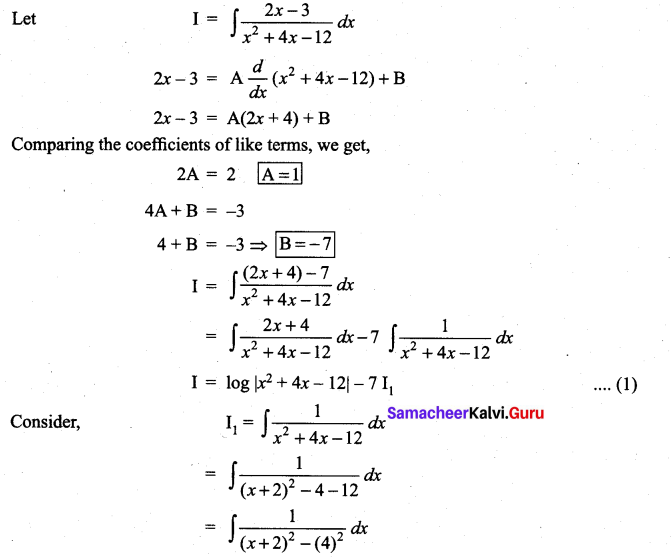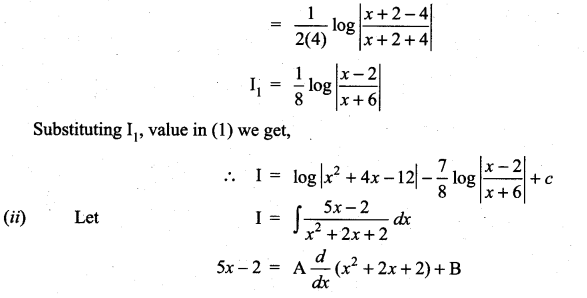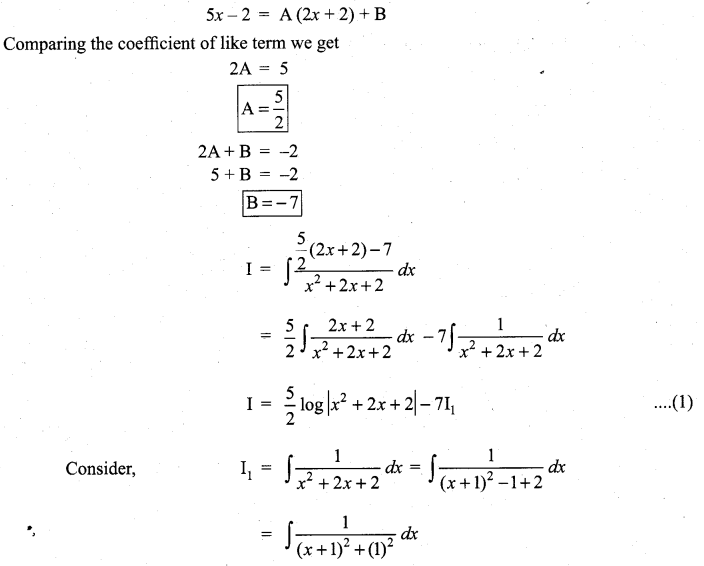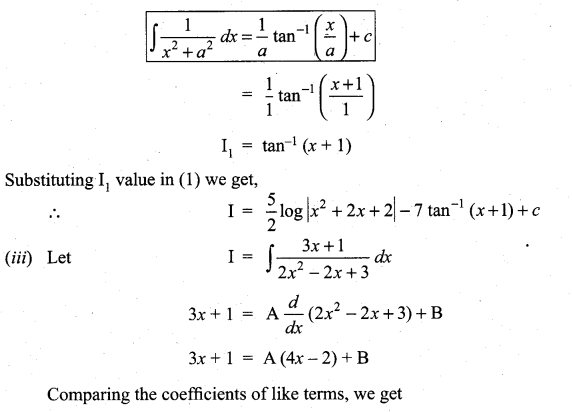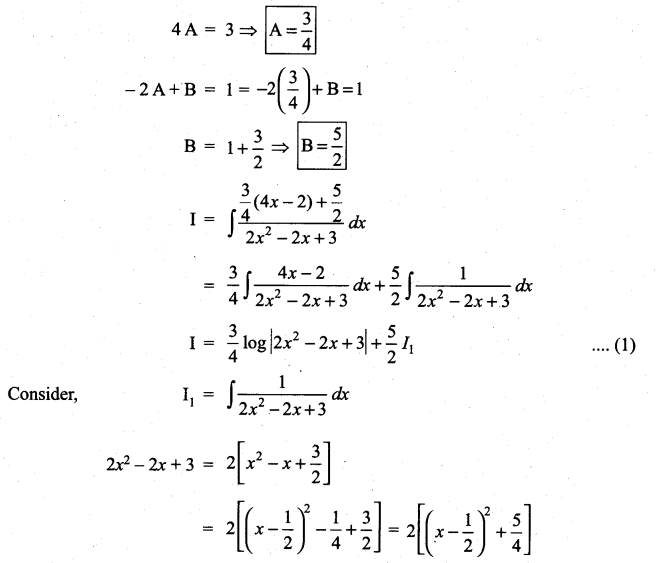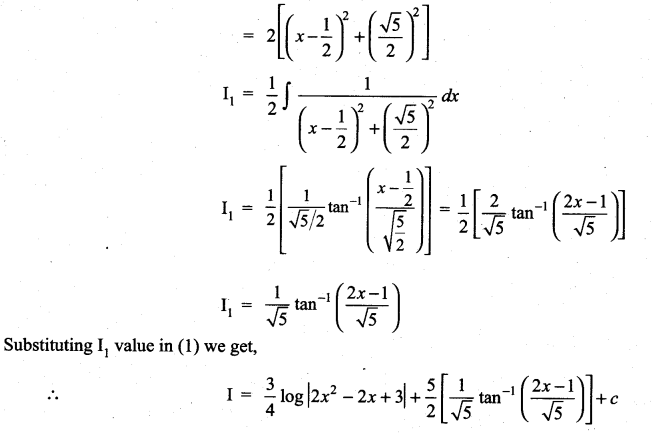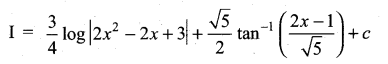Question 2.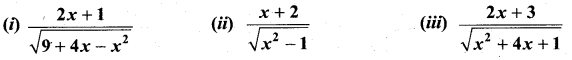Solution: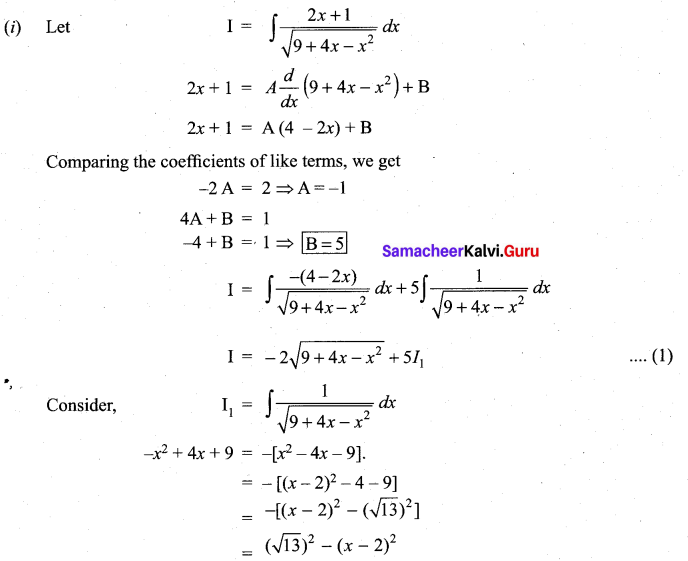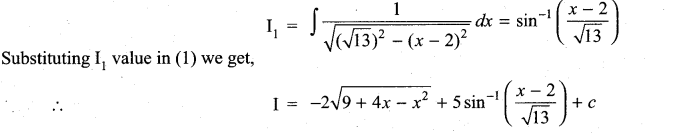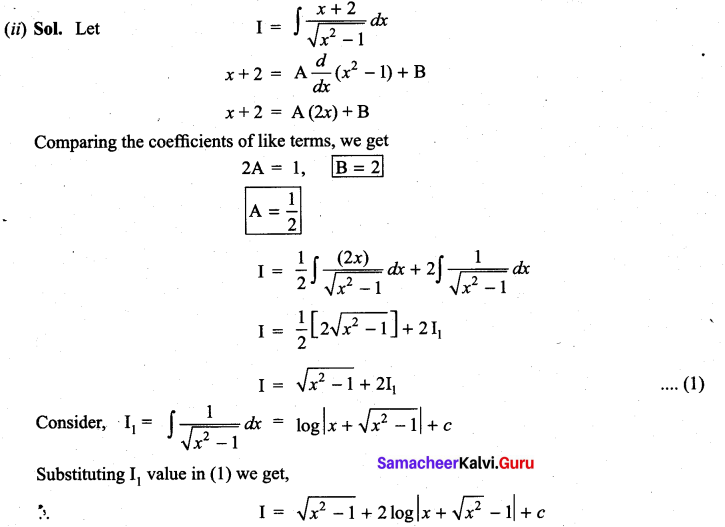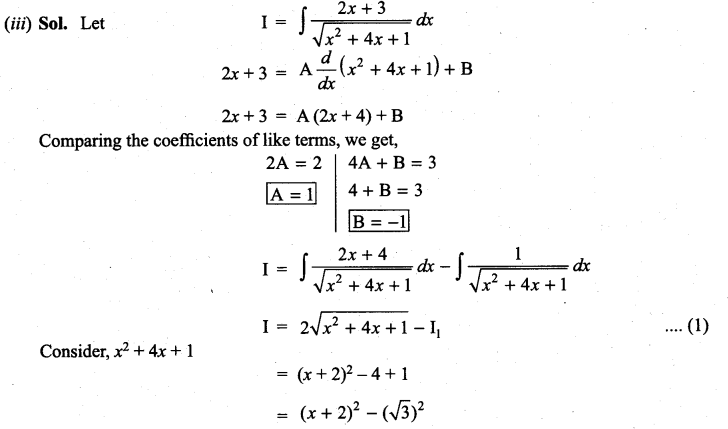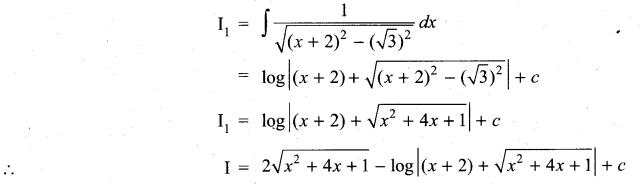### Samacheer Kalvi 11th Maths Solutions Chapter 11 Integral Calculus Ex 11.11 Additional Problems

Question 1.
$$\frac{2 x-1}{2 x^{2}+x+3}$$
Solution: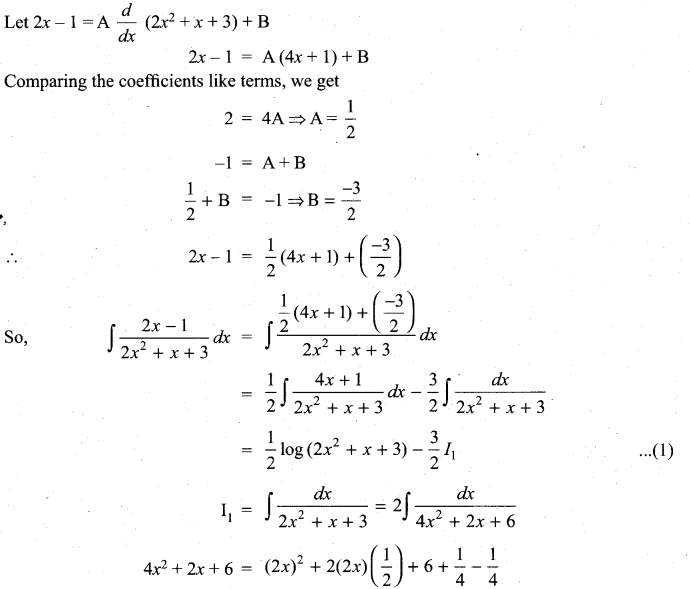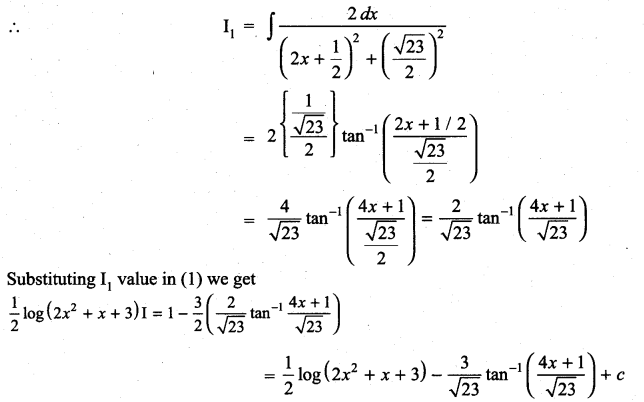Question 2.
$$\frac{4 x+1}{x^{2}+3 x+1}$$
Solution: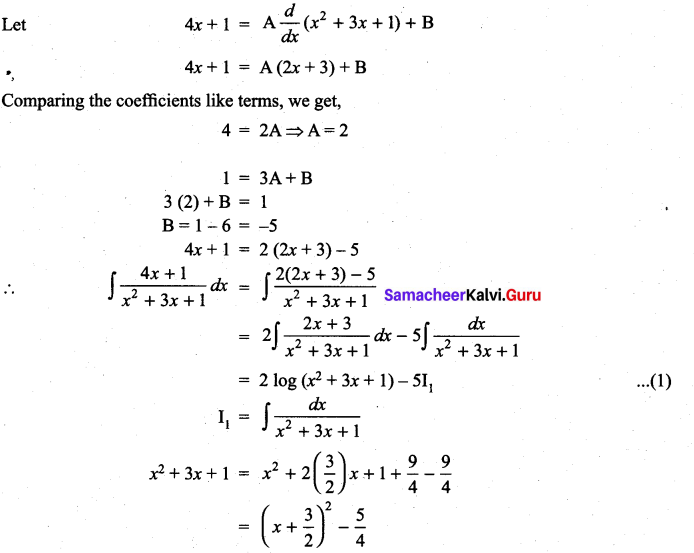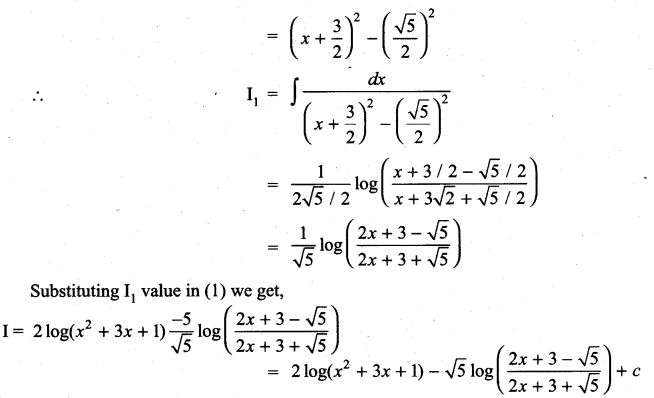Question 3.
$$\frac{2 x-3}{\sqrt{10-7 x-x^{2}}}$$
Solution: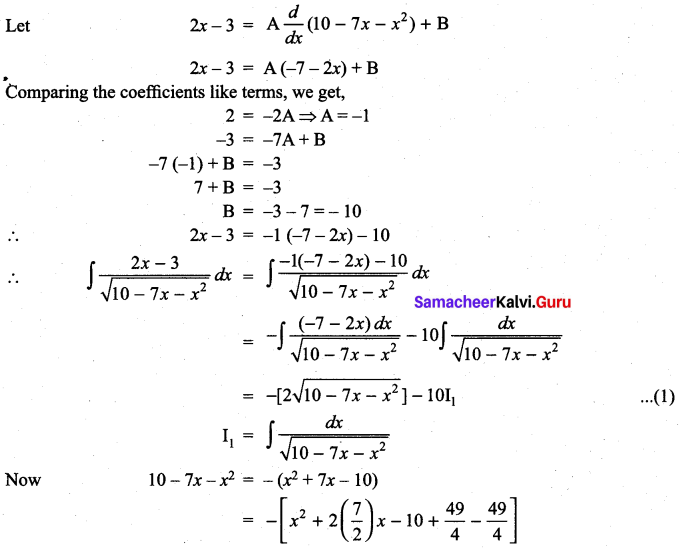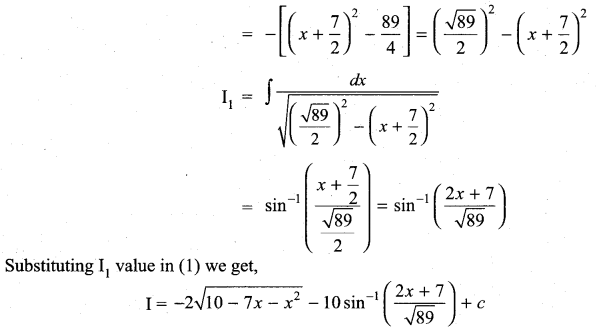Question 4.
$$\frac{6 x+7}{\sqrt{(x-4)(x-5)}}$$
Solution: